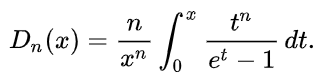# Debye Function

The Debye function (also called the Debye scattering function or the Debye Integral) is an uncommon special function, first developed by Peter Debye in the early 20th century during his studies in heat capacity.

Debye’s model was developed to estimate the phonon contribution (a phonon is basically a particle of heat) to a solid’s heat capacity. The model treats atomic vibrations as phonons in a box (i.e. a solid), in contrast to Einstein’s model which considers a solid as a collection of non-interacting quantum harmonic oscillators. Debye’s model performs well at low temperatures, while Einstein’s does not.

Debye functions are now used in a wide range of related fields including nanoscience, solid state physics, spectroscopy, and thermodynamics.

## Formula for the Debye Function

The Debye function formula is:Where n—a non negative integer— is the order of the function.
Dn and tn tell you the order of the function:

• D1 / t = first order,
• D2 / t2 = second order,
• D3 / t3 = third order,
• D4 / t4 = fourth order,
• D5 / t5 = fifth order,
• D6 / t6 = sixth order.

The Debye functions are notoriously difficult to work with by hand, partly due to the definite integral included in the formula; Wunderlich (2005) calls the simplest one-dimensional Debye function “rather complicated”. However, most popular mathematical software (e.g. R) have built in or add on functions to work the formula. That said, tables for numerical evaluation are widely available, including those listed in this pdf.

## References

Abramowitz, M. and Stegun, I. A. (Eds.). “Debye Functions.” §27.1 in Handbook of Mathematical Functions with Formulas, Graphs, and Mathematical Tables, 9th printing. New York: Dover, p. 998, 1972.
Beyerlein, K. (2013). A review of Debye Function Analysis. Retrieved January 23, 2020 from: https://bib-pubdb1.desy.de/record/167429/files/DESY-2014-01781.pdf
Chem Europe. Debye Model. Retrieved January 23, 2019 from: https://www.chemeurope.com/en/encyclopedia/Debye_model.html
Numerical Evaluation of Debye Function. Retrieved January 23, 2020 from: http://duffy.princeton.edu/sites/default/files/pdfs/links/Debye_Function.pdf
Wunderlich, B. (2005). Thermal Analysis of Polymeric Materials. Springer Science & Business Media.

CITE THIS AS:
Stephanie Glen. "Debye Function" From StatisticsHowTo.com: Elementary Statistics for the rest of us! https://www.statisticshowto.com/debye-function/